Automation, Control and Intelligent Systems
Volume 4, Issue 1, February 2016, Pages: 1-9

Data-Driven Models and Methodologies to Optimize Production Schedules

Prabhakar Sastri*, Andreas Stephanides

Automation and Data Analytics Department, Isa Technologies Pvt. Ltd., Manipal, India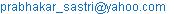(P. Sastri)

Prabhakar Sastri, Andreas Stephanides. Data-Driven Models and Methodologies to Optimize Production Schedules. Automation, Control and Intelligent Systems. Vol. 4, No. 1, 2016, pp. 1-9. doi: 10.11648/j.acis.20160401.11

Abstract: Data driven Models based on production parameters in combination with modern optimization algorithms are shown to be useful in industry to optimize production schedules and improve profitability. Based on real data obtained from an existing facility, we have developed models for time and costs of heat treatment. Using these data statistical models have been developed and used to find an optimal solution to the Job-Shop scheduling problem using three algorithms namely Particle Filter, Particle Swarm Optimization and Genetic Algorithm. The algorithm is useful when we would like to arrive at job schedules based on a mix of both time and cost optimization. The results are compared and future work discussed with respect to the data used.

Keywords: Data-Driven Models, Optimized Production Scheduling, Job-Shop Scheduling, Time Based and/or Cost Based Production Optimization, Management Decision Tool

Contents

1. Introduction and Background

A heat treatment facility typically consists of many furnaces. The parts undergoing heat treatment can be processed in one or more furnaces. Each furnace can also process a variety of parts. A decision on price and resource allocation has to be made every time a part gets processed in a facility consisting of many furnaces. The problem becomes more complicated if only partial processing can be done in a furnace and hence a part may have to be processed in more than one furnace.

Delivery of some parts is time critical and others are cost critical. There are tactical and operational level decisions to be addressed including the cost of a single job, which may be optimized either for cost or time. Based on the type of individual jobs to be processed, it is also necessary to optimize the overall profitability of the facility and hence work out the production schedule and resource allocation.

Imagine a scenario:

A set of jobs have been booked from various clients. Some are "time critical" and the others "cost critical". Once a list of such jobs is available, it becomes necessary to work out a production schedule which accommodates all these jobs based on the current loading pattern in the furnaces of the facility.

It needs to be reiterated that

Each furnace can process many different types of parts.

It is possible to process multiple parts in a furnace at the same time. (Currently this is outside the scope of the present paper).

It may be possible / necessary to process a part in either one furnace or a combination of furnaces.

The cost of processing of the parts is different in each of the furnaces.

In our case the two main parameters to decide which resources should be used at what time are duration and cost of a job. The priorities may vary for each job. We optimize these parameters depending on the preferences for a set of jobs.

The optimal solution for a production schedule depends not only on the preferences regarding cost and duration, but also on the estimation of these parameters based on the furnace in which they are processed.

The objective of this paper is to present an algorithmic methodology of solving resource allocation and scheduling problem. The methodology takes into account that the model for cost vs time optimization in various furnaces is data driven i.e. based on the data collected from existing furnaces and the jobs processed so far. The need to update the model also depends on the number of furnaces. If the number of furnaces or the numbers of type of parts processed changes very often then it may be required to update the model more frequently. This is also true if the type of parts are different from the one based on which the model was created. This could vary from once a day to several times a month. The data driven model is generic enough to be applied to any facility and once the parameters are estimated, the model can be used only for that facility.

The paper is organized as follows:

In the second section we give a review of the literature and the work done so far to understand the importance of the current work.

In the third section we formulate empirical Models for duration and costs based on data provided by an existing facility in India.

In the fourth section we start with optimizing a single resource allocation decision and extend that to take into account multiple jobs to be scheduled.

The optimization problem becomes a variation of a Job-Shop Scheduling problem. These problems were first described in Graham . Dimopoulos  compared different research about Job-Shop Scheduling problems and showed that recent research increasingly takes into account costs and other optimization criteria that are more relevant to real manufacturing decisions. Since we optimize a real live production facility we will consider multiple parameters for our optimization.

Finally we explore future work.

2. Review of Previous Work

The Job-Shop Scheduling problem is known to be n-p hard. Different algorithms are used to solve these problems. Mathirajan, Chandru and Sivakumar  discuss in detail the process of heat treatment and the operations prior to that of preparation of molten metal and its casting. They optimized the operation of two furnaces using heuristic algorithms. Their assumptions include the following:

a  Due to technical reasons, it is not possible to process jobs from different families together in the same batch. We shall call these job-families incompatible. Furthermore, these jobs will have to be processed without interruption on parallel and non-identical BPs (BPs with different capacities), which are available continuously with an objective of maximizing the utilization of the BPs.

b  Scheduling planning period is one week

c  All batch processors are continuously available and all jobs must pass through the operation(s) to be carried out at the BPs.

They successfully used data from an existing foundry and suggested that "the way forward would be to be able to schedule the jobs at more frequent intervals than the current 24 hrs that they used as jobs arrive in the shop floor at shorter intervals".

Amin Jamili & Mohammad Ali Shafia & Reza Tavakkoli-Moghaddam  proposed a hybrid algorithm based on particle swarm optimization and simulated annealing for a periodic job shop scheduling problem. They suggest that "evolutionary algorithms are finding more use than those for various reasons such as convergence speed". They compare the various algorithms and point out that PSO has the advantage of memory: the characteristics of the good solutions are retained by all particles even if the population has changed etc.

Recently Gomez Urrutia, Aggoune and Dauzere-Peres  used heuristics to solve lot-sizing and scheduling problems. The scheduling of the tasks is an operational one whereas the need to satisfy the objective of cost and time is a tactical one and the two cannot be pursued independently. We close that gap and integrate preferences for a single job in the tactical production plan as well as using the production plan to decide on facility optimization measures.

Dimopoulos  has extensively reviewed research using advanced optimization methods to solve scheduling problems in production. Particle Swarm Optimization (PSO) and Genetic Algorithms (GA) are widely used. Luarn  compared PSO to GA. Jamili, Shafia and Tavakkoli-Moghaddam  combined PSO with simulated annealing. Genetic algorithms are used by Wang, Yin and Wang  and by Pfund  for the scheduling problem.

For these reasons, we have considered Particle Filter, Particle Swarm Optimization and Genetic Algorithm for our work.

We introduce an approach to solve the optimization problem, using three commonly used methods. These are Particle Filter, Particle Swarm Optimization and Genetic Algorithm referred to above. We use random-key representation as described by Bean  of the priority of the jobs and a scheduling rule to get a robust representation that is independent of the optimization algorithm used. The main advantage of this approach is that compared to most heuristics, parameters by which the optimal decision is influenced is very clear.

3. Models for Duration and Costs

We begin by formulating the Model based on the data collected from the automation system installed in the plant.

3.1. Empirical Models for Duration

To formulate a Model for the duration of heat treatment, data from an existing facility has been used. The facility has 20 furnaces and processes about 109 different parts. The types of furnaces include Sealed Quench Furnaces, Pit Furnaces, Nitriding Furnaces, Rotary Hearth and other furnaces. The data is over a period of 22 months.

Before a detailed statistical analysis is possible, the data has to be cleaned. For the current scenario the following was done for the following reasons:

Missing fixture weights were added: This is to ensure that fixtures which also get heated in the furnace and hence require time and energy are considered.

Charges which are involved with rework were removed. This is to ensure purity of data as reworking would mean quality of the end product is not acceptable.

Errors and missing values in part weights were fixed: This is to ensure that the data is correct. The part weight is available in the plant database.

Charges that have been processed incompletely were removed.

Gross weight with fixed values was recalculated.

Durations for each process step were calculated.

Charges with more than one part were removed, and

Only charges processed completely in automatic mode were considered.

After cleaning the data, a total of 557 batches with 109 different part numbers from 10 different furnaces have been analyzed.

To increase the data used for the model, the quality of the stored data is very important. To implement our framework one should consider optimization of the collected data. In the next phase of the work, it is planned to include data from a larger number of furnaces over a larger period of time with more number of parts. It is necessary to emphasize that quality and quantity are both critical and understanding this would further strengthen the usefulness of this work.

We give a brief review of the data here.

Figure 1 shows the process steps.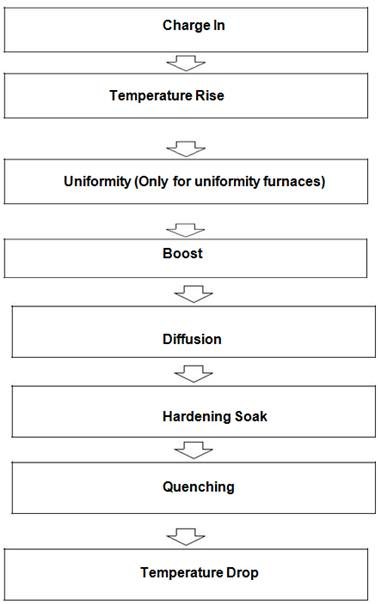Figure 1. Process Steps.

It is not necessary that all the steps be followed when processing a job. To give an idea of the complexity of the data, we present below the duration graphs of reach of the stages described above. We may mention that we are willing to provide the raw data to anyone interested as it would enable a better understanding of the Modeling possible and different viewpoints will always be welcome. The data will be in Microsoft Excel format.

The graphs depict the variety of processes taking place in the furnaces and the many complex ways of handling and heat treating various parts.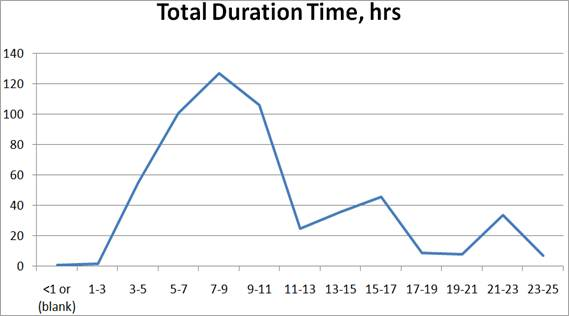Figure 2. Distribution of Total Duration (557 data points).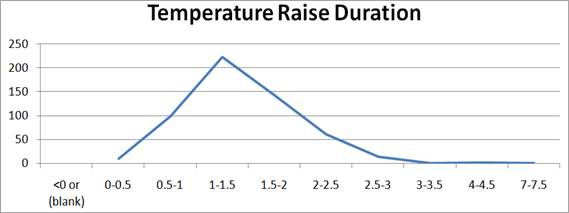Figure 3. Distribution of Temperature Rise Duration (557 data points).Figure 4. Distribution of Uniformity Duration (378 data points, blanks & zero omitted).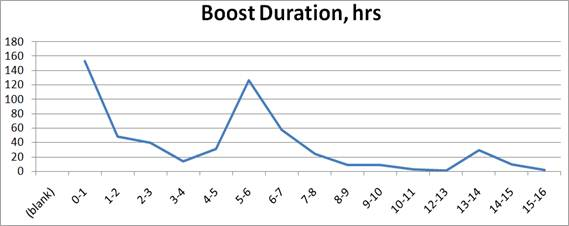Figure 5. Distribution of Boost Duration (487 data points, less than 3 minutes omitted).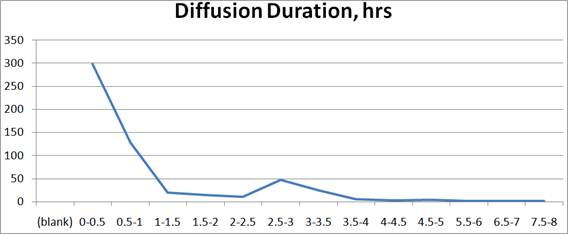Figure 6. Distribution of Diffusion Duration (327 data points, less than 10 minutes omitted).Figure 7. Distribution of Hardening Soak Duration (411 data points, less than 10 minutes omitted).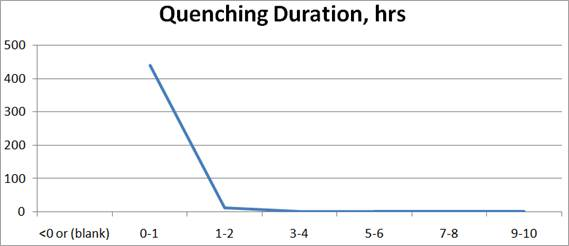Figure 8. Distribution of Quenching Duration (454 data points, less than 5 minutes omitted).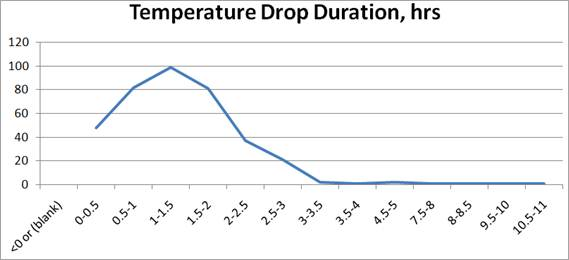Figure 9. Distribution of Temperature Drop Duration (377 data points, blanks omitted).

We may mention here that we have been collecting data from this facility and hope to have a much larger and varied data from more number of furnaces now connected to the system processing many more different parts. The Models developed by us continue to be used and updated regularly but is limited at present to the furnaces considered above. We hope to have in place a system which will permit automatic updating of the Models presented here based on the data collected.

3.1.1. Model I

On this cleaned dataset a statistical analysis of the duration of the process was done. We proposed a linear Model with the gross weight as parameter and an offset for each furnace. The Model I for the duration TI (Total Time for processing the job) can be written as

TI = c + cF + cgw GW,  (1)

with a constant general offset c, a offset for a furnace F, cF and the parameter for the influence of the gross weight GW, cgw.

Table 1. Statistical Analysis of Model I: Tests of Between-Subjects Effects.

 Dependent Variable: Duration Source Sum of Squares df Mean Square F Corrected Model 18842.484a 20 942.124 43.07 Intercept 9082.692 1 9082.692 415.3 Gross Weight 1450.404 1 1450.404 66.31 Furnace 14613.46 19 769.13 35.16 Error 74017.08 3384 21.873 Total 495362 3405 Corrected Total 92859.56 3404

a. R Squared =.203 (Adjusted R Squared =.198)

Table 1 shows the statistical analysis of the general linear Model I. It can be seen, that each of the factors are statistically significant for the Model.

Since the fit of a statistical, linear model always includes uncertainties, the 95% confidence interval of the fitted parameters is considered. With those, an optimistic and a pessimistic time estimate is achieved and used as a quality criterion. Since the Model is to be used for resource allocation decisions and cost calculation, the uncertainty should be quantified. The resulting interval turns out to be typically around +/- 3 hours for the Model I.

3.1.2. Model II

The detailed model includes applying the method of Model I but for each individual process step shown in figure 1.

For each of these process steps, the model can be written as

Tp = c + cFp  (2)

where c is for the entire facility and cFp is a function of the furnace and the process step. The time for "charge in" is not considered here, because in our data it is zero in most cases or close to it. This could be significant is other facilities and can then be considered for such facilities.

Uniformity time is only considered if it is a uniformity furnace. Model II for the duration TII can be written as

TII = ∑ Tp  (3)

with the duration of process step i, TII;i. The estimates for the process steps and the parameters used in the test case are given in tables 2 and 3. The results are summarized in Table 4. This shows that for the detailed Model II the interval between the optimistic and the pessimistic estimate is larger, because the uncertainties of the parameters for each step add up and result in a more conservative estimation of the interval.

Table 2. Process step estimates, parameters as in Table 3.

 Temperature Raise 01:28:03 Uniformity 00:41:54 Boost 08:45:43 Diffusion 00:32:08 Hardening Soak 00:03:05 Quenching 00:33:46 Temperature Drop - 0:01:35 Total Duration 12:06:13 ~12:15:00

Table 2 shows the estimates on the process steps for a test case. It can be seen, that the negative estimate for the temperature drop is not an acceptable value. The value becomes negative because of the linear Model and the confidence interval and has to be interpreted as zero or close to zero estimates and the uncertainty on these estimates should be considered. For the overall estimate the values should not be removed from the calculation. Since we considered the uncertainties in Model II to be too high to use this Model in the scheduling task and we did not actually need estimates of each process step, we did not investigate this any further. However larger amount of data over a variety of conditions would reduce these uncertainties and make this Model more useful.

Table 3. Parameter for Test case.

 Parameter Value Gross Weight 880kg Part No HM127415XD Furnace 10X

3.1.3. Model III

Finally we investigate a Model including the part number (Model III), it can be written as

TIII = c + cF+ cpartno + cgw GW  (4)

where cpartno is the offset for each part number.

In this model the validity of the estimate is expected to be higher, because it takes into account the offset of a specific part. The main disadvantage is that the confidence interval of the parameters is higher, because the data per part is considerably smaller. To improve this model, more data will have to be collected.

3.1.4. Comparison of the 3 Models

Table 4 shows that all three Models are consistent and lead to similar estimates. The difference is the size of the intervals between optimistic and pessimistic estimates. Model I has the closest interval that can be explained by the different amount of data used in the Model to arrive at the estimates. The more detailed the Model gets, the less data can be used to estimate a certain parameter. Model III takes into account the specific part numbers and some parts have been processed very rarely. A larger set of data can lower these differences.

Table 4. Estimates for Test Case, parameters as in Table 3.

 Estimated Duration Pessimistic Optimistic Model I 12:45:00 15:15:00 09:57:39 Model II 12:15:00 16:45:00 07:15:00 Model III 12:45:00 26:15:00 06:00:00

If table 5 is compared with table 4 it will be noticed that the historical data for a certain part number is consistent with the estimates of all three Models. It should however be considered, that this will not be true for a single observation because even the expected interval allows outliers. Due to the high uncertainties and the similarity of the estimates, it was decided to use the simplest Model. We therefore use Model I (equation 1) for the scheduling.

Table 5. Historical data, parameters as in table 3.

 Average duration 12:27:31 No cases 23

This gives us a direction for future work. If the data is continuously collected and upgraded, it is possible to execute the Model at a regular interval and as the volume of data grows larger the parameters are expected to be estimated better.

3.2. Model for Cost

Some costs are easily quantifiable but it is hard to assign them to a single charge. Therefore the average tonnage of each furnace is used to calculate a per weight markup m for each furnace. Costs per hour can be included using the average hours per month. We used data from the same facility to calibrate our Model.

costs(F, GW ) = m(LF, X, C, fcF) GW  (5)

with the parameters described in table 6.

Table 6. Parameters in Cost Model.

 Parameter Description F Furnace LF Labour costs of furnace C Capital costs for furnace X Fixture costs fcF Fuel costs per month

4. Optimal Production Schedule

In this section we show how an optimal production schedule can be generated, based on a Model for cost and duration. We use Model I equation (1) with slight modifications for the duration and equation (5) for costs. Although we depend on the models developed here, they can be replaced by any other suitable model.

4.1. Single Decision Step

Before we approach the overall optimization of the scheduling problem we first consider a single decision for scheduling one job.

4.1.1. Simple Optimization Problem

So far we described Models for duration and costs for a single charge. On these we can base a decision assuming that all or a given set of furnaces are available. With this proposition we can find the optimal solution based on costs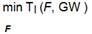(6)

or duration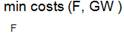(7)

One can also find an optimal solution regarding costs with a constraint to duration,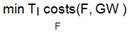(8)

costs(F, GW ) < costsmax

or an optimal solution regarding duration with a constraint to cost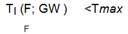(9)

The chances are that the furnaces may not be available for certain time due to preoccupation with different jobs. To consider this, one can add a schedule that takes previous decisions into account. We thus consider previous scheduled jobs to be constraints to the optimization problem. This can be achieved by calculating the waiting time for each furnace and add it to the duration.

TA(F, GW ) = TI (F, GW ) + Twait(F)  (10)

Table 7. Parameters for cost function.

 Parameter Value d;j 0 …. 100a c;j 1/4000 pend 1000 penc 1000 (1/4000)^2

a. In almost every case 0

4.1.3. Multi-parameter Penalty Function

In this step we extend the optimization problem to include costs and duration at the same time along with the constraints. For the constraints we use the penalty method to relax the constraints and include them in the objective function.

We introduce a new objective function, which includes duration and cost for each job weighted by the preferences for the job. For one Job j the objective is to find the solution to

min JF

F

with

JF =wd;j TA;j(F;GW)+ wc;j costs(F;GW)+ pend (TA;j(F;GW) - Tmax;j)2+ penc (costs I;j(F;GW) - costmax;j)2  (11)

with pend = 0 if the duration including waiting time is smaller than the maximum duration and penc = 0 if costs are smaller than the maximum costs and otherwise according to table 7, wd;j is the weight of duration for job j and wc;j the weight of costs for job j, these weights should be chosen much smaller than the weight of the penalty function and relative to each other according to preferences for each job. Note that here we use the extended duration model given by (10) and the cost model (2). Since the preferences for each job are different we take into account, that for some jobs the duration is critical and for some the duration is not. The advantage of dropping the assumption that preferences are the same for every job is that it reflects the real economic situations. The resulting schedule is optimized by the priorities of each job and tries to meet as many constraints as possible.

4.2. Multiple Job Scheduling

We now schedule a list of jobs in an optimal way. In our model we can neither assume that each job will have the same parameters on each furnace nor that the decision criteria will be the same for each job. Equation (9) shows that the total penalty is the sum of the penalty for each job, by using the scheduling rule the problem can be reduced to finding an optimal order to schedule the jobs.

We propose as a scheduling rule, that if we have the jobs in an ordered list we always take the optimal single decision regarding the penalty function (8) for every job. It can be argued, that for a global optimum a non optimal decision for a single job can only be accepted if the benefits of the optimal decision for another job are higher. If that is the case the job which would benefit more should be prioritized, i.e. being scheduled first. We propose as a scheduling rule, that if we have the jobs in a ordered list we always take the optimal single decision regarding the penalty function (11) for every job.

That for optimization a prioritized list can be transformed into a schedule by schedule builders is used in many studies as shown by Dimopoulos  To represent the order of the list we will use random-key representation as described in Bean  and Uzsoy 

The overall objective is to find a solution to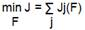(12)

By these rules we get a schedule that optimizes (12). Due to the random-key representation of the ordered list, we have a very strong representation that leads for every set of random keys to a feasible solution. We are able to use any optimization algorithm to find the optimal set of random-keys, which corresponds to an optimal schedule.

In some papers related to operation scheduling, heuristics are used to find a schedule. The argument is usually that the problem is N-P hard, e.g. in Mathirajan, Chandru, and Sivakumar  a heuristic for steel industry was developed. As already mentioned in section 1 on page 1, there are also a lot of references proposing genetic algorithms and particle swarm optimization to solve a Job-Shop problem.

We compare the advanced optimization techniques: particle filter, particle swarm optimization and a genetic algorithm, with the goal to find an optimal schedule. A set of jobs similar to existing data is used and certain restrictions are assumed. Taillard  proposed certain benchmarks for their optimization, since the main goal is to solve our specific problem the focus is on that problem.

4.2.1. Particle Filter (PF)

Each ordered list of jobs is considered a particle, with all scheduling information attached. The particle filter has with genetic algorithm in common, that both algorithms can be used to solve highly non-linear optimization problems if the penalty function can be calculated easily, but the gradient can't.

The particle filter selects the best particles and modifies them randomly. This can be achieved by adding a random number r to the key of each job in the list. The random number should be smaller than the initial interval to reduce the modification. The descendants of a particle should be similar to the particle; the optimal variation of the particle depends on how close the particle is to the optimal solution.

We propose to choose 21 particles and keep the best, particle 0, unchanged, modify particles 1-10 and remove particles 11-20. Particles 11-20 are replaced by descendants of the best particles.

The particle filter uses algorithm 1, with the random modification operator RM(l; σ), which modifies each key of the jobs in list l by a random variable with a normal probability distribution with a variance σ.

Algorithm 1 Schedule optimization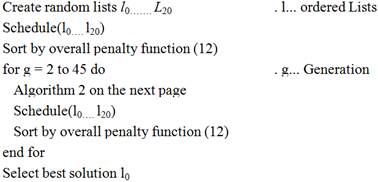4.2.2. Genetic Algorithm (GA)

The genetic algorithm can be understood as an extension of the idea of particle filters. The algorithm 1 is modified by replacing algorithm 2 by algorithm 3. We retain the number of generations and the overall structure to make it comparable. For industry usage, the generations should be chosen dependent on the selected algorithm and the necessary convergence.

We use a statistical multi-point crossover operator (MXO) with a 0.05 probability to crossover after each gene. Therefore the points for the crossover are random and the number of crossovers is random.

Algorithm 2 change ordered lists by particle filter

for i=11 to 14 do

li çRM(l0; 0.1)
end for

for i=15 to 16 do

liçRM(l1; 0.5)

end for

for i=17 to 18 do

liç RM(l2; 0.6)

end for

for i=19 to 20 do

li çRM(l3; 0.6)

end for

for i=1 to 10 do

li RM(li; 0.5)

end for

Genetic algorithms are known to be useful to solve multi-objective problems and are widely used in context of realistic scheduling problems in manufacturing; Shaw et al  used genetic algorithms to solve multi-objective scheduling problems in batch-processing.

Algorithm 3 change ordered lists by genetic algorithm

for i=11 to 14 do

j   rand(1..10)

li = MXO(l0; lj)

RM(li; 0:01)

end for

for i=15 to 16 do

j  rand(1..10)

li = MXO(l1; lj)

RM(li; 0:1)

end for

for i=17 to 18 do

j  rand(1..10)

li = MXO(l2; lj)

RM(li; 0:1)

end for

for i=19 to 20 do

j  rand(1..10)

li = MXO(l3; lj)

RM(li; 0:1)

end for

for i=1 to 10 do

RM(li; 0:4)

end for

4.2.3. Particle Swarm Optimization (PSO)

In Jamili, Shafia, and Tavakkoli-Moghaddam  Wang, Yin, and Wang ) and many similar studies, Particle Swarm Optimization is used to solve Job-Shop scheduling problems. Jamili, Shafia, and Tavakkoli-Moghaddam  combine it with simulated annealing to a hybrid optimization process. Particle swarm optimization is inspired by the behavior of animal swarms. Each particle has a speed and uses it's own best known solution and the swarms best known solution to update its speed. The keys of each job can be collected in a vector k(li), the speed of the particle v(li) is the change rate of the keys. The keys of generation G + 1 of a particle i, kG+1(li) are calculated by

kG+1(li) = kG(li) + vG+1(li)   (13)

The speed vG+1(li) is

vG+1(li) = wa vG(li) + wb r + wc (kG(li) bG(li))+ wd (kG(li)- bG(l0…….l20)) (14)

with the weights wj, a random vector r, bG(lj…….lk), the best known key set of lists j to k.

Algorithm 4 change ordered lists by particle swarm optimization

for i=0 to 20 do

r   rand()

Update speed (11)

Update particle key (10)

end for

4.3. Results

To compare the different algorithms we compiled a set of 75 jobs with different constraints, preferences, part number and weight. These jobs were to be scheduled in an optimal way using the different optimization algorithms. To make the results comparable we always used the same number of generations. The weights of the penalty functions are chosen as in table 7. Figure 10 shows that the three tested algorithms all converge to a result within the given amount of generations. The found optimum is in all cases a different one. GA found on average a better optimum than the other algorithms.

It must be noted that all the three algorithms gave similar results and one is free to choose the algorithm to use.

Thus the methodology provides an elegant process of generating the production schedule for the scenarios described in page 1.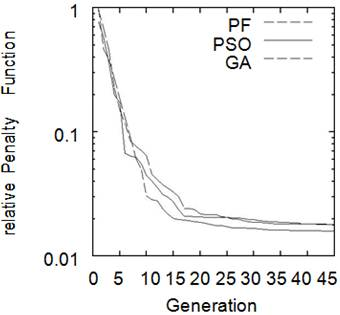Figure 10. Comparison of convergence of different algorithms.

5. Conclusions and Outlook

The framework can be used in several ways. The most obvious is to get an optimal production schedule based on models driven by real production data. The models could be improved by using additional data or parameters in the Models for duration and costs. We showed in section 4.1.3 how to optimize a single scheduling decision for one job and in section 4.2 how to extend that to an optimal schedule. The Model is currently in use at the facility from which the data has been taken but is generic enough to be used at other facilities. Efforts are on to install and get similar data from another facility for the same purpose.

It must be mentioned that the method and models are generic. They can be applied to any facility. Since the data will be different, the model parameters will be decidedly different and hence the schedules will also be different. We will also mention that the models need periodic updating. The frequency depends on the changes brought about in the facility. If the changes are not many, the results are not expected to be very different. On the other hand if frequent additions/changes are made it is necessary to update the model regularly. The judgment is left to the facility manager. It is extremely easy to rework the model as the algorithm is coded in an Excel sheet to and just adding/ changing data and sending a request is adequate to update the model. The data can be directly linked to the automation system as has been done by us and this makes it easier to update the model.

We tested multiple optimization algorithms for that and found that these algorithms can be utilized to solve real life optimization problems in industry. There are other possible applications of our framework and we leave these as future work.

6. Future Work

Only a few possible applications for our framework have been shown. It could be extended to answer other questions in industry regarding resource allocation decisions. In section 3.1.4 it was decided to use Model I. The scheduling could also be investigated with Model II or Model III.

A possible improvement is to develop more detailed Models for duration and costs of the process, including extended data of furnaces, parts or the production process.

Additionally the framework could be easily tested in a real live environment with a greater set of jobs and preferences.

References

1. Graham, Ronald L. Bounds for certain multiprocessing anomalies. Bell System Technical Journal 1966; 45 (9): 1563-1581.
2. Dimopoulos, A.M.S., C.; Zalzala. Recent developments in evolutionary computation for manufacturing optimization: problems, solutions, and comparisons. IEEE Transactions on Evolutionary Computation 2000; 4.
3. Mathirajan, M, V Chandru, and AI Sivakumar. Heuristic algorithms for scheduling heat-treatment furnaces of steel casting industries. Sadhana 2007; 32 (5): 479-500.
4. Jamili, Amin, Mohammad Ali Shaa, and Reza Tavakkoli-Moghaddam. A hybrid algorithm based on particle swarm optimization and simulated annealing for a periodic job shop scheduling problem. The International Journal of Advanced Manufacturing Technology 2011; 54 (1-4): 309-322.
5. Gomez Urrutia, Edwin David, Riad Aggoune, and Stephane Dauzere-Peres. Solving the integrated lot-sizing and job-shop scheduling problem. International Journal of Production Research (ahead-of-print): 2014; 1-19.
6. Luarn, Ching-Jong Liao; Chao-Tang Tseng; Pin. A discrete version of particle swarm optimization for flowshop scheduling problems. Computers & Operations Research 2007; 34.
7. Wang, Yong Ming, Hong Li Yin, and Jiang Wang. "Genetic algorithm with new encoding scheme for job shop scheduling."The International Journal of Advanced Manufacturing Technology 2009; 44 (9-10) 977-984.
8. Pfund, Lars Monch, Hari Balasubramanian; John W. Fowler; Michele E. Heuristic scheduling of jobs on parallel batch machines with incompatible job families and unequal ready times. Computers & Operations Research 2005; 32.
9. Bean, James C. Genetic algorithms and random keys for sequencing and optimization. ORSA journal on computing 1994; 6 (2): 154-160.
10. Uzsoy, Cheng-Shuo Wang; Reha. A genetic algorithm to minimize maximum lateness on a batch processing machine. Computers & Operations Research 2002; 29.
11. Taillard, E. Benchmarks for basic scheduling problems. European Journal of Operational Research 1993; 64.
12. Shaw, KJ, AL Nortcli e, M Thompson, J Love, PJ Fleming, and CM Fonseca. Assessing the performance of multiobjective genetic algorithms for optimization of a batch process scheduling problem. Proceedings of the 1999 Congress on Evolutionary Computation, 1999; CEC 99, Vol. 1.

 Contents 1. 2. 3. 3.1. 3.2. 4. 4.1. 4.2. 4.3. 5. 6.
Article ToolsAbstractPDF(1457K)